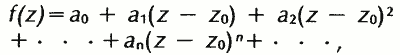# complex variable analysis

## complex variable analysis,

branch of mathematicsmathematics,
deductive study of numbers, geometry, and various abstract constructs, or structures; the latter often "abstract" the features common to several models derived from the empirical, or applied, sciences, although many emerge from purely mathematical or logical
that deals with the calculuscalculus,
branch of mathematics that studies continuously changing quantities. The calculus is characterized by the use of infinite processes, involving passage to a limit—the notion of tending toward, or approaching, an ultimate value.
of functions of a complex variable, i.e., a variable of the form z=x+iy, where x and y are real and i=√−1 (see numbernumber,
entity describing the magnitude or position of a mathematical object or extensions of these concepts. The Natural Numbers

Cardinal numbers describe the size of a collection of objects; two such collections have the same (cardinal) number of objects if their